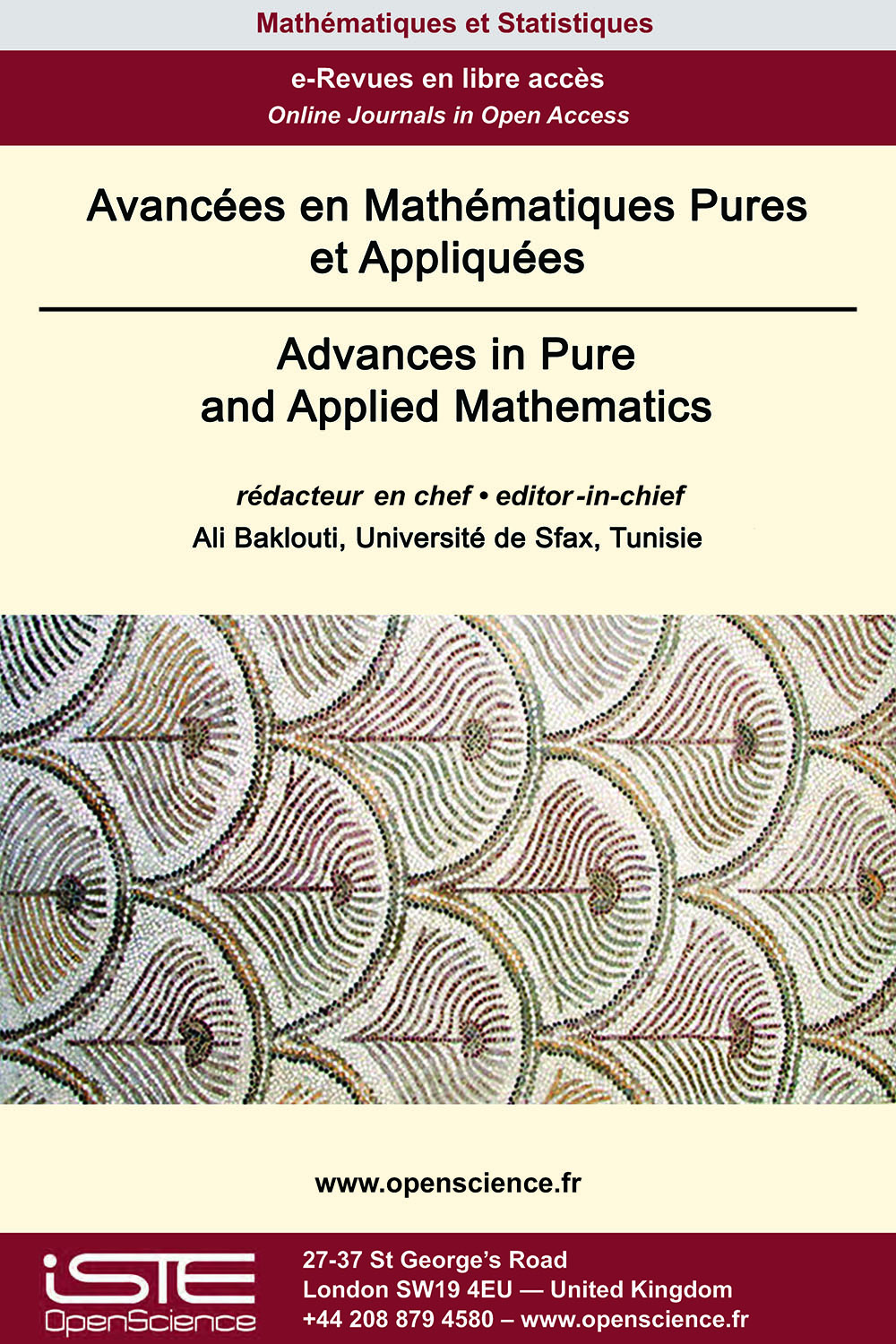# Vol 13 - Numéro 1 (Janvier 2022)

## Articles parus

Contrôle optimal d’une diffusion fractionnée Problème de Sturm-Liouville sur un graphe étoile

This paper is devoted to parabolic fractional boundary value problems involving fractional derivative of Sturm-Liouville type. We investigate the existence and uniqueness results on an open bounded real interval, prove the existence of solutions to a quadratic boundary optimal control problem and provide a characterization via optimality system. We then investigate the analogous problems for a parabolic fractional Sturm-Liouville problem on a star graph with mixed Dirichlet and Neumann boundary controls.

Opérateurs de composition pondérés symétriques complexes sur un espace de Hardy à poids

In this paper various conditions under which a weighted composition operator $W_{\psi,\phi}$ on the weighted Hardy space $H^2(\beta)$ becomes complex symmetric with respect to some special conjugation have been explored. We also investigate some important properties of the complex symmetric operator $W_{\psi,\phi}$ such as hermiticity and isometry.

Théorème du point fixe de type Krasnoselskii dans les espaces de Banach ordonnés et application aux équations intégrales

In this paper, we present a variant of Krasnoselskii’s fixed point theorem in the case of ordered Banach spaces, where the order is generated by a normal and minihedral cone. In such a structure, there is a possibility to give a new sence to the concept of contraction.

Terracini Loci et espaces homogènes

We study the linear dependence of disjoint unions of double points of an integral and non-degenerate variety $X\subset ℙ^r$. Such sets are called Terracini loci. Our main results are for Segre-Veronese embeddings and a few other homogeneous spaces. To study the minimal number of such double points which are linearly dependent, it is useful to study the minimal degree curves contained in $X$. We give an example (the Segre embedding of ℙ1$\times$ ℙ1) in which these curves are not suffcient to describe these Terracini loci.### Autres numéros :

2020

Volume 20- 11

Numéro 1 (Mai 2020)
Numéro 2 (Septembre 2020)

2021

Volume 21- 12

Numéro 1 (Janvier 2021)
Numéro 2 (Mai 2021)
Numéro spécial : AUS-ICMS 2020
Numéro 3 (Septembre 2021)

2022

Volume 22- 13

À paraître

Numéro 1 (Janvier 2022)
Numéro 2 (Mars 2022)